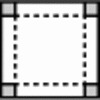#### You may also like### Bracelets

Investigate the different shaped bracelets you could make from 18 different spherical beads. How do they compare if you use 24 beads?### Sweets in a Box

How many different shaped boxes can you design for 36 sweets in one layer? Can you arrange the sweets so that no sweets of the same colour are next to each other in any direction?### Making Boxes

Cut differently-sized square corners from a square piece of paper to make boxes without lids. Do they all have the same volume?

# Pebbles

##### Age 7 to 11Challenge Level

A group of children from Manorfield Primary School, Stoney Stanton sent in lots of ideas:

S.B. and N.L. produced the following table of results:

 Number Shape Number of pebbles on side Area of shape Perimeter of shape 1 Square 2x2 1cm$^2$ 4cm 2 Rectangle 2x3 2cm$^2$ 6cm 3 Square 3x3 4cm$^2$ 8cm 4 Rectangle 3x5 8cm$^2$ 12cm 5 Square 5x5 16cm$^2$ 16cm 6 Rectangle 5x9 32cm$^2$ 24cm 7 Square 9x9 64cm$^2$ 32cm 8 Rectangle 9x17 128cm$^2$ 48cm

PATTERNS AND FORMULAE
It was said in whole class discussion that the pattern for the size of the shapes was:
Squares: each side is the same length as the longest side of previous rectangle
Rectangle: one side is the same length as the side of the previous square, the other side is a "new length"

A.H and E.R also said:

The formula for the area = $2$ to the power of $(n-1)$.
The pattern for the new side of the rectangles is $+1$, $+2$, $+4$, $+8$, $+16$ ... (it doubles)
The pattern for the perimeter is $+2+2$, $+4+4$, $+8+8$, $+16+16$ ... (it doubles)

Now, Alice from St Thomas' Church of England Primary School, wrote;

On B (how many pebbles there are round the edges), the pattern starts on 4 adds 2 which makes  6 adds another 2 which is eight then adds 4 which makes 12 adds 4 again (16) adds 8  (24) adds 8 adds 16, and so on.
So it adds one thing twice then doubles the adding number and starts to do that again.

Amelia from NLCS sent in her ideas;

First double forward then double sideways and so on